Mathematical and Physical Journal
for High Schools
Issued by the MATFUND Foundation
 Already signed up? New to KöMaL?# Exercises and problems in InformaticsMay 2002

I. 25. According to Zeckendorf's theorem, every natural number n can uniquely be expressed as a sum of Fibonacci numbers n=Fk1+Fk2+...+Fkr such that ki$\displaystyle \ge$ki+1+2 and kr$\displaystyle \ge$2 hold for all i (1i<r), where, as usual, Fibonacci numbers satisfy F0=0, F1=1 and Fk=Fk-1+Fk-2 (k>1).

Given a natural number n (1n107), your program (I25.pas,...) should decompose it into a sum of Fibonacci numbers. (Efficiency of your solution will also be taken into account.) (10 points)

I. 26. Let us consider a square. The Sierpinski carpet is obtained by recursively taking off smaller and smaller squares: at the first level the middle square with side being one-third of the original one is removed. Then, in the second level, divide the remaining area into 8 smaller squares and repeat the previous process again. The remaining 8

.8 squares (with area being one-ninth of the previous squares each) are handled in the same way in the third level, and so on.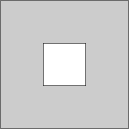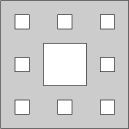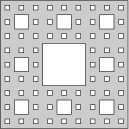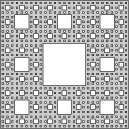level 1 level 2 level 3 level 4

Prepare your program (I26.pas,...) which reads the number of the level, and draws the corresponding Sierpinski carpet. (Parts that have not been removed should be coloured grey.) (10 points)

I. 27. The French flag problem is the following control problem. N adjacent cells (operating in the same way) should be equipped with and appropriate state-transition function (that is a formula to be written into the cell) such that the cells form a French flag pattern as a result (i.e. N/3 cells become red, N/3 cells become white and N/3 cells become blue).

In the initial state, on disturbing the leftmost cell, 3 waves (i,j,k) emerge from it. The i-wave stays for 1 time step in each cell before moving to its right neighbour. The j-wave stays for 2, while the k-wave stays for 5 time steps in a cell before moving to the next one. The i-wave is reflected from the rightmost cell and annihilates each of the waves it encounters.

The original grey colour of a cell changes according to the type of the wave: i-waves give blue, j-waves give white and k-waves give red colour to the cells they pass over. If a cell meets a reflected i-wave, its colour is unchanged in the future.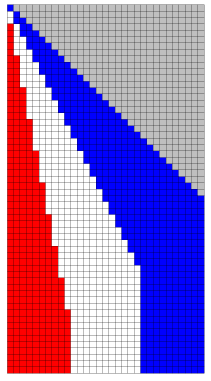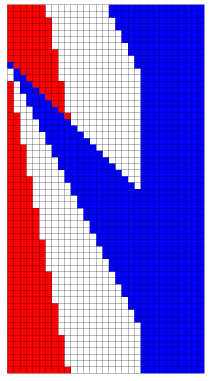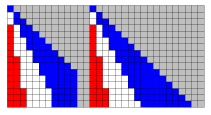Figure 1 Figure 2 Figure 3

If the leftmost cell is disturbed once more, then it initiates i-, j- and k-waves, and the pattern is formed again (see Figure 2). Finally, if the row of cells is pulled apart (that is an empty column is inserted), then the pattern will appear in both parts (see Figure 3). (Figures are on the bottom page of this paper.) Prepare an Excel sheet (I27.XLS) that solves the French flag problem. (10 points)